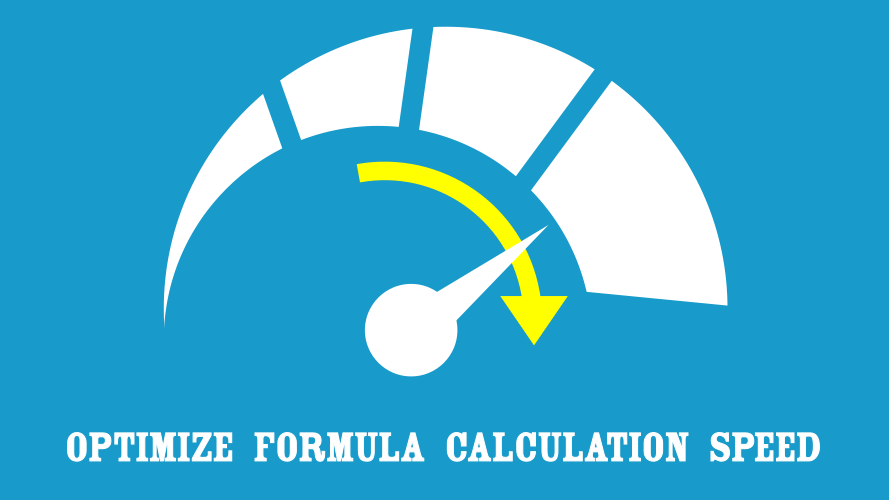# Optimize Formula Calculation Speed – Part 10: Summary## Part 10: Summary

In this series, we have undertaken a variety of tests to identify what will provide us with the fastest calculating formulas.  Now it is time to bring all of those learnings together.  In this final part, we will try to focus on implementing the methods which will give us the greatest benefit.

## Single criteria

Where there is a single criterion the following will help to reduce calculation times:

• Use VLOOKUP or INDEX/MATCH for single criteria lookups (Part 4 – Lookup formulas)
• Sort data only where the results looked up are weighted towards the records earlier in the source data set.  Do not sort data unless you have tested that it saves calculation time, as it is also possible for sorting data to slow down a calculation.  We need to consider this on a case by case basis (Part 5 – Sorting source data).  (This assumes we are not using an approximate match method).
• Summarized data with unique records calculate faster than source data which requires a calculation (SUMIF for example) to be performed.   Therefore, if it is possible to summarize the data to the right levels first it can significantly reduce calculation time. (Part 4 – Lookup formulas compared with Part 8 – Sum with multiple criteria)

## Multiple criteria

Where there are multiple criteria the following will reduce calculation times:

## Other factors

Many other factors have a much smaller impact.  Focus on getting the formula and data layout right first, then consider other items which could be fine-tuned.

## Other resources

There are other resources which may also give you ideas about how to optimize formula calculation speed.

## 8 thoughts on “Optimize Formula Calculation Speed – Part 10: Summary”

1.Will Hayes says:

This was fantastic. Thanks for posting.

•Excel Off The Grid says:

Hi Will,

Thank you for the comment. I appreciate the support and I’m glad you found the post useful. Hopefully this has saved you some time (there is nothing more frustrating that watching that blue wheel spin round and round).

2.Daryl L says:

Hi – Have you considered comparing these results to Get & Transform / Data Model / DAX which have become available recently in Excel.

•Excel Off The Grid says:

Microsoft have re-developed the Excel Calculation engine recently. It should soon be rolled out to all 365 subscribers. At that point, I my refresh these posts as the calculation times are likely to change.

Having to click Refresh make Get & Transform a more ‘manual’ type calculation. Though, using the CUBE functions would be an interesting comparison, I might do that.

3.rdm128 says:

Thank you for this. It helped my cut recalc times on a revenue model from 20 minutes to 3 minutes. Still not near adequate, but much easier for me to keep at it.

The referenced timers do not work on 64 bit excel. As I’m not a developer, any hint on the changes needed to get them going?

•Excel Off The Grid says:

Try this:

```' Test whether you are using the 64-bit version of Office 2010.
#If VBA7 Then
Private Declare PtrSafe Function getFrequency Lib "kernel32" _
Alias "QueryPerformanceFrequency" (cyFrequency As Currency) As Long

Private Declare PtrSafe Function getTickCount Lib "kernel32" _
Alias "QueryPerformanceCounter" (cyTickCount As Currency) As Long
#Else
Private Declare Function getFrequency Lib "kernel32" _
Alias "QueryPerformanceFrequency" (cyFrequency As Currency) As Long

Private Declare Function getTickCount Lib "kernel32" _
Alias "QueryPerformanceCounter" (cyTickCount As Currency) As Long
#End If
```
4.Ben Sandford says:

Very helpful article! What about DSUM? How does that compare with SUM (Array) or SUMIFS? And does the new addition of “@” syntax change anything?

•Excel Off The Grid says:

The Excel team have made some changes to the calculation engine about 12 months ago. This will impact all of this series, so I will need to re-write much of this.

In terms of DSUM, it’s not a common enough function to include in the scope of this post.